# Graphs of Straight LinesPage 1

#### WATCH ALL SLIDES

Slide 1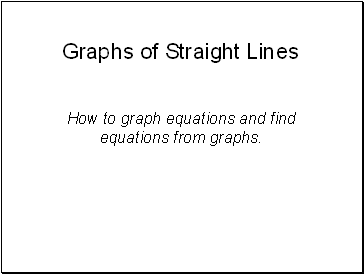## Graphs of Straight Lines

How to graph equations and find equations from graphs.

Slide 2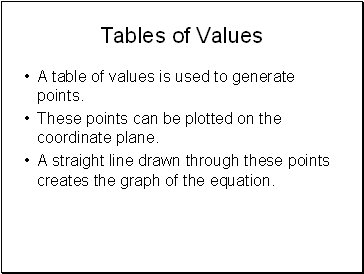## Tables of Values

A table of values is used to generate points.

These points can be plotted on the coordinate plane.

A straight line drawn through these points creates the graph of the equation.

Slide 3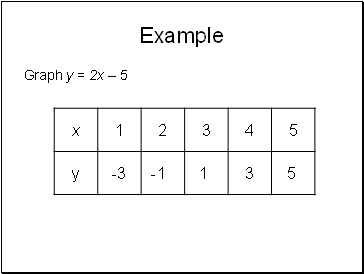Example

Graph y = 2x – 5

-3

-1

1

3

5

Slide 4Plot these points on the coordinate plane.

Slide 5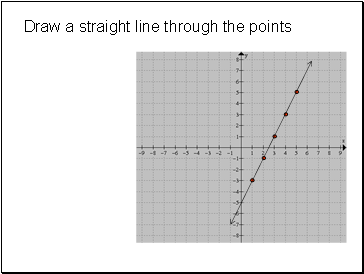## Draw a straight line through the points

Slide 6Exercises

Graph the following straight lines:

y = 3x – 4

y = 2x + 1

y = x – 5

y = -3x +2

Look for a relationship between the graphs and their algebraic expressions!!!

Slide 7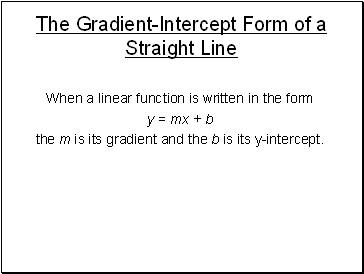## The Gradient-Intercept Form of a Straight Line

When a linear function is written in the form

y = mx + b

the m is its gradient and the b is its y-intercept.

Slide 8## What’s the Equation?

For the following graphs, determine their equation.

Slide 9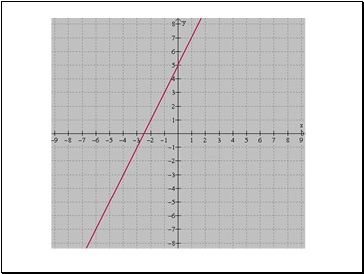Slide 10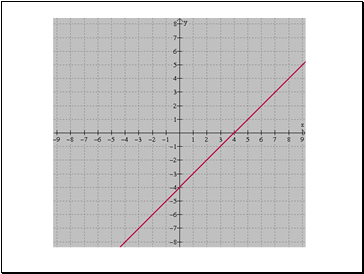Slide 11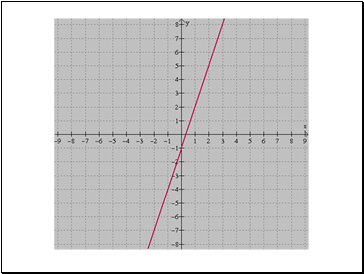Slide 12Slide 13